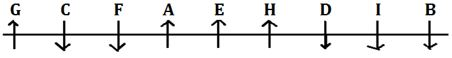Latest Banking jobs   »

# Reasoning Ability Quiz For IBPS PO Prelims 2022- 16th September

Directions (1-5): Study the following arrangement carefully and answer the questions given below.

9 D H 8 I % T @ I J M Q 3 © R 4 P W \$ Y 2 A E 6 # U K 5 U 1 & Z N

Q1. Which of the following element is the seventh to the left of the fifteenth from the left end of the given arrangement?
(a) I
(b) T
(c) @
(d) J
(e) None of these

Q2. How many such numbers are there in the given arrangement, each of which is immediately preceded by a consonant and immediately followed by a vowel?
(a) None
(b) One
(c) Two
(d) Three
(e) More than three

Q3. How many such symbols are there in the given arrangement, each of which is immediately followed by a letter and immediately preceded by a number?
(a) None
(b) One
(c) Two
(d) Three
(e) More than three

Q4. How many such consonants are there in the given arrangement, each of which is immediately preceded by a consonant and immediately followed by a symbol?
(a) None
(b) One
(c) Two
(d) Three
(e) More than three

Q5. Which of the following element is 18th from right end of the series?
(a) 4
(b) R
(c) P
(e) None of these

Directions (6-10): Study the following information carefully and answer the given questions:
Nine students A, B, C, D, E, F, G, H and I are sit¬ting in a straight line equidistant from each other, but not necessarily in the same order. Some of them are facing south while some are facing north.
F sits third from one of the extreme ends of the row and facing same direction as I but opposite to H. More than three persons sits between B and F. A sits second to the left of the one who sits third to the right of B who is facing south. Four persons sit between C and D both are facing same direction as B. Neither A nor B is an immediate neighbour of C and D. Both immediate neighbour of E facing same direction. H is not immediate neighbor of C. D sits left of C. I sits third to the right of E, neither I nor E immediate neighbour of F. Only three persons sit between G and E and both are facing same direction.

Q6. Who among the following sits third to the left of E?
(a) A
(b) B
(c) C
(d) D
(e) F

Q7. How many persons sits between F and the one who sits second right of I?
(a) None
(b) two
(c) three
(d) one
(e) four

Q8. How many persons are facing south direction?
(a) Two
(b) Three
(c) Five
(d) One
(e) Four

Q9. What is the position of D with respect of F?
(a) Fourth to the left
(b) Immediate right
(c) Fifth to the right
(d) Immediate Left
(e) Third to the right

Q10. Who among the following sits third from the left end?
(a) E
(b) G
(c) C
(d) A
(e) F

Directions (11-15): Study the following arrangement carefully and answer the questions given below:

N I J 5 ¥ 3 F H 8 U B 6 @ W L 2 © K Q # G % T 9 7 A P \$ € E D

Q11. How many such symbols are there in the above arrangement, each of which is immediately preceded by a consonant but not immediately followed by a number?
(a) None
(b) One
(c) Two
(d) Three
(e) More than three

Q12. Four of the following five are alike in a certain way based on their positions in the above arrangement and so form a group. Which is the one that does not belong to that group?
(a) J¥5
(b) 8BU
(d) QG#
(e) T79

Q13. Which of the following is the 9th to the right of the 20th from the right end of the above arrangement?
(a) G
(b) %
(c) 2
(d) K
(e) @

Q14. If the last twelve elements in the above arrangement are written in the reverse order, then which of the following will be the twenty-fourth from the left end?
(a) A
(b) P
(c) \$
(d) 9
(e) T

Q15. How many such consonants are there in the above arrangement, each of which is immediately preceded by a number and immediately followed by a symbol?
(a) None
(b) One
(c) Two
(d) Three
(e) More than three

Solutions

S1. Ans. (c)

S2. Ans. (d)
Sol. H8I, Y2A, K5U

S3. Ans. (d)
Sol. 3©R, 6#U, 1&Z

S4. Ans. (b)
Sol. PW\$

S5. Ans. (a)

Solutions (6-10):
Sol.S6. Ans. (c)

S7. Ans. (b)

S8. Ans. (c)

S9. Ans. (a)

S10. Ans. (e)

S11. Ans. (d)
Sol. Q#, G%, P\$

S12. Ans. (c)

S13. Ans. (a)

S14. Ans. (b)

S15. Ans. (a)#### Congratulations!Download Hindu Review of October 2021: Free PDF